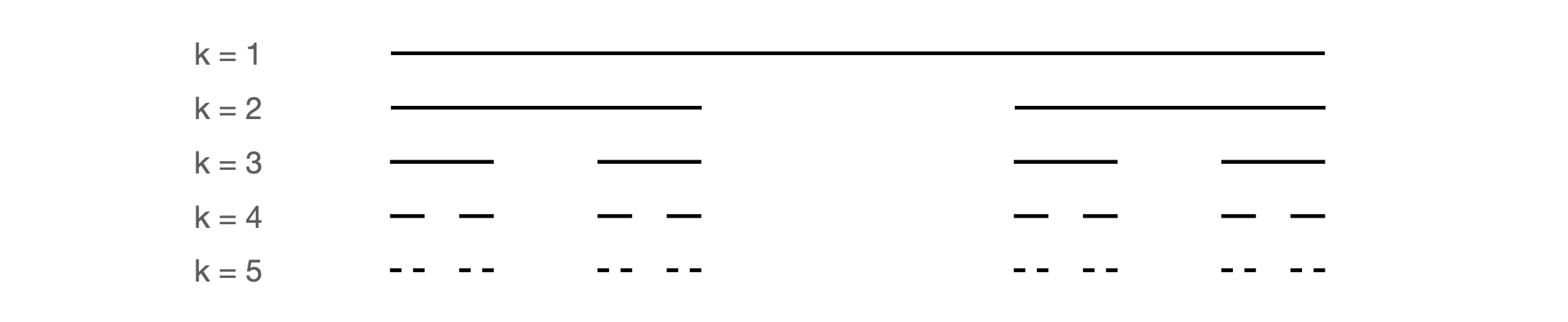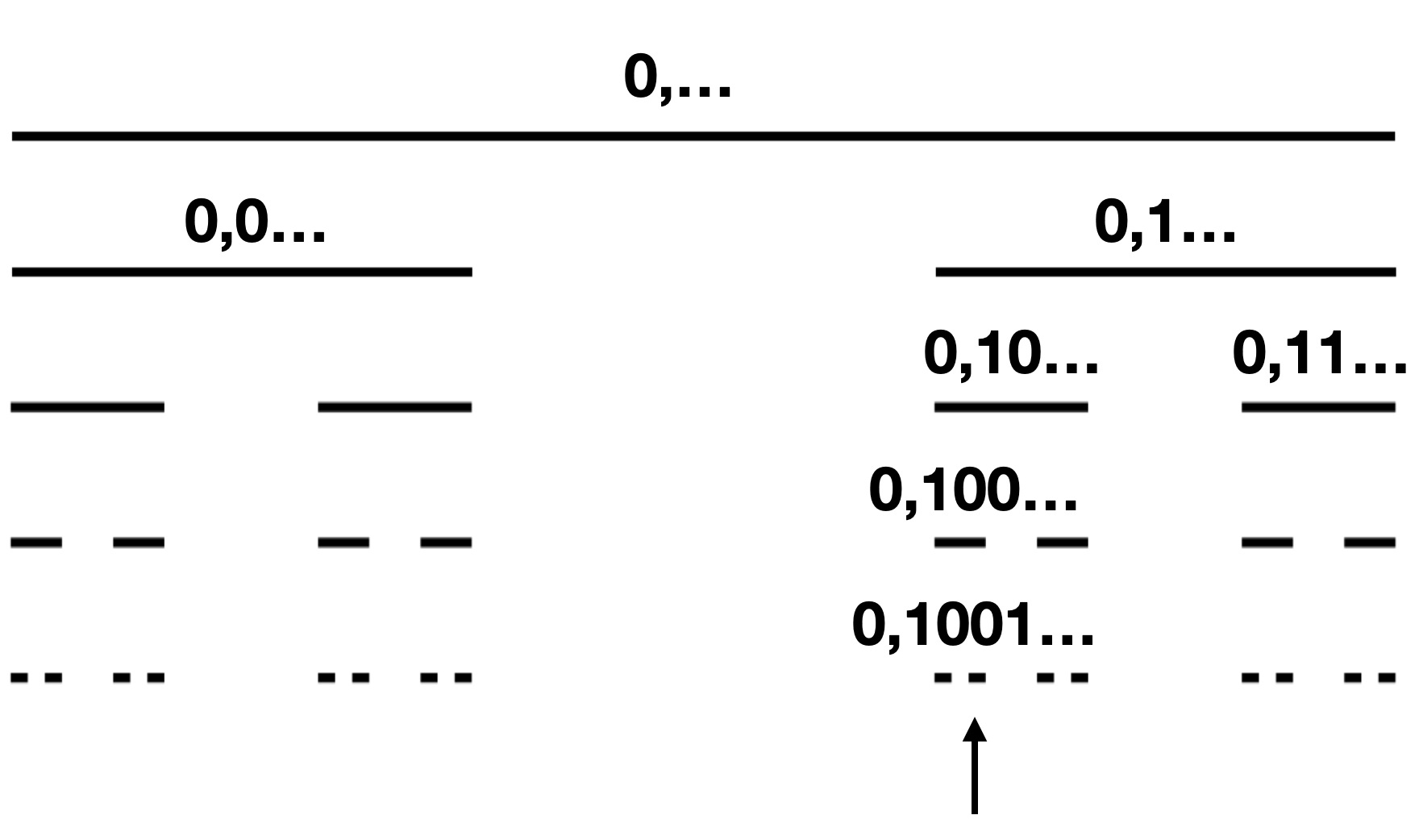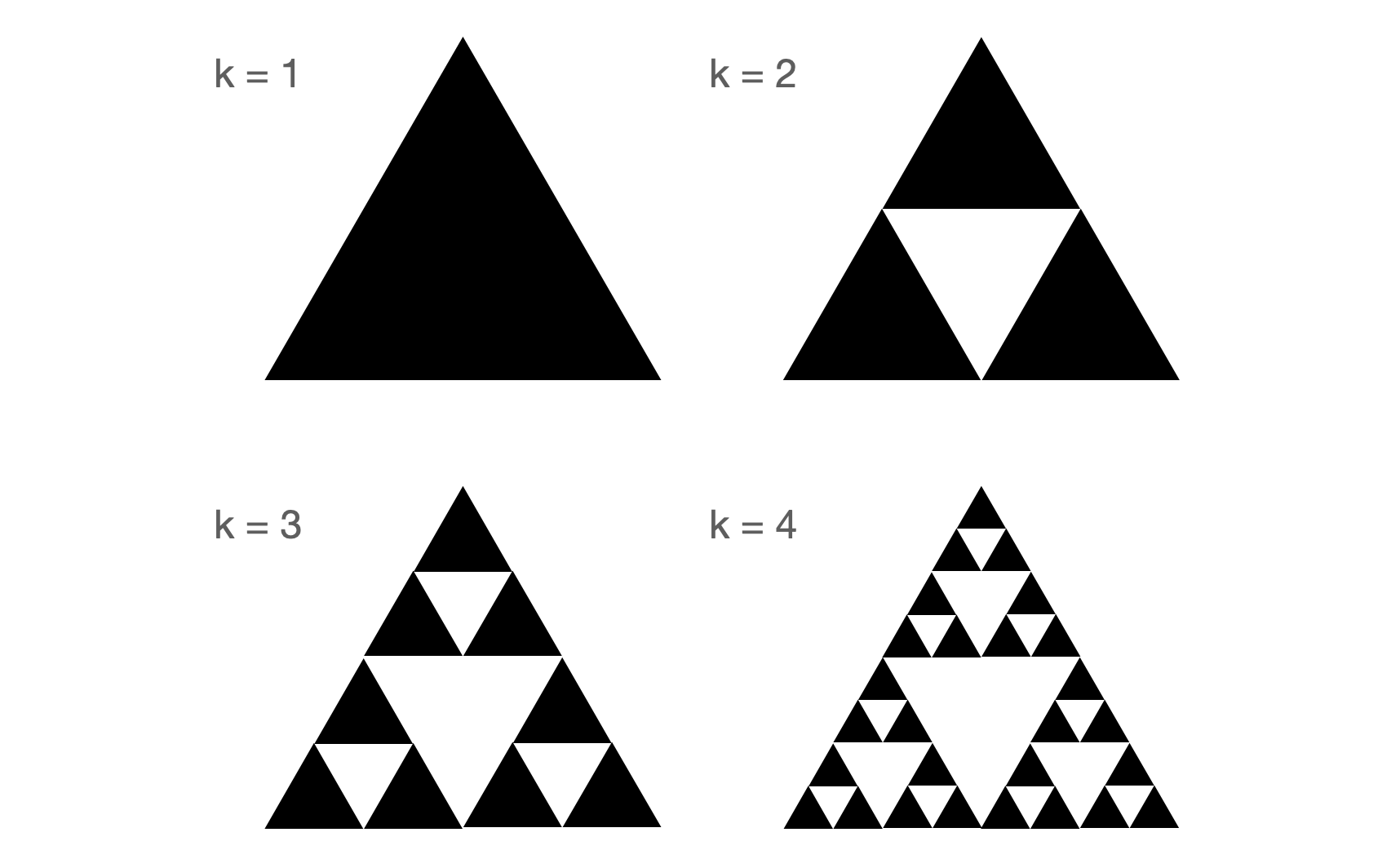## 1.2 Hello, fractals!

It’s fascinating that an endless repetition of the same actions can lead to the creation of fascinating figures – fractals.

Anyone can draw such a figure with some basic knowledge of programming. We will show how to construct different classes of fractals step by step. Knowledge of the basics of algebra, probability and topology will allow you to understand more precisely where these surprising figures come from.

### 1.2.1 Cantor dust

One of the more interesting methods of constructing fractals is the method `by removal’. We take a particular shape and then remove pieces from it. What is left is a fractal, often with surprising properties. Let’s illustrate this with an example of a fractal called (Cantor dust()) after Georg Cantor, a pioneer of set theory, who described the Cantor set in 1883.

Recipe for Cantor dust construction.

1. Take a line segment of any length (but for simplicity, ours will be of length 1).
2. Divide this line segment into three equal parts.
3. Remove the inside of the middle part, which will give you two line segments, both of length 1/3 of the original line segment.
4. For each resulting line segment, continue dividing by returning to step 2.

For an illustration of five consecutive steps of the biting algorithm, see Figure 2.Figure 2: First 5 iterations of Cantor dust construction

The above algorithm is characterized by several elements typical for fractals. First, it never ends; the whole procedure must (at least in theory) be repeated infinitely many times. Second, we are dealing with recursion. Two objects are created as the result of the third step, and then each of them is transformed again in the same way as the initial object.

Proceeding in a very similar way, you can obtain many interesting figures, but let’s look at Cantor dust for a little bit longer. Let’s see what we actually obtained as a result of this procedure.

Let’s check how big the object is, that is, what is its length. Initially, the line segment had a length of $$1$$, but in the first step, we removed $$1/3$$ of it. In the second step, we removed $$(1/3)^2$$ twice. Repeating this several times, in step $$k$$, we removed $$2^{k-1}$$ line segments, each of length $$(1/3)^k$$. So the length of this creation after step $$k$$ is:

$\begin{equation} \begin{split} 1 &- 1/3 - 2*(1/3)^2 - ... - 2^{k-1}*(1/3)^k = \\ & 1 - \sum_{i=1}^k 2^{i-1}*(1/3)^i = 1 - 1/2 \sum_{i=1}^k (2/3)^i = \\ & 1 - \frac 12 (2 - 2 (2/3^k)) = (2/3)^k \xrightarrow[k\rightarrow \infty]{} 0 \end{split} \end{equation}$

We can reach the same conclusion using a different way reasoning, namely by looking at how much is left of a segment after the $$k$$-th step. After the first step, we have $$2$$ line segments of length $$1/3$$, after the second step, we have $$2^2$$ line segments of length $$(1/3)^2$$, and after the $$k$$-th step, we have $$2^k$$ line segments of length $$(1/3)^k$$. What does this sequence converge to?

$\lim_{k \rightarrow \infty} (2/3)^k = 0$

There is no doubt. The Cantor set has a length equal to 0. But clearly, it is not an empty set because it has many points. How many? It turns out that as many as the whole line segment, and therefore uncountably many. Let’s show this using a clever proof.

Theorem (Cardinality of Cantor set). Cantor set is equinumerous with the interva $$[0, 1]$$.

Proof: In order to count the points in the Cantor set, we must construct a representation for each point, that is, a notation that allows us to identify each point uniquely. A representation that uniquely identifies a point will be the set of decisions determining how to reach that point in the subsequent steps of the procedure that generates Cantor dust.

We remember that in each step, the centers are removed from the line segments, so the point that belongs to Cantor dust will lie either in the left or the right part of the line segment. This choice (left/right) must be made in each step of the dust construction. We can write such a representation as an infinite sequence of 0/1 digits – if 0 occurs in the sequence at a position $$k$$, the point belongs to the left subsegment, and if 1, it belongs to the right subsegment.Note the unambiguity -> any point from Cantor dust can be described by an infinite sequence of 0/1 digits. At the same time, each infinite sequence of digits describes some point from Cantor set, and different sequences represent different points. How many such sequences are there? As many as in the whole line segment. Just think of these sequences as binary expansions of the numbers in the interval $$[0, 1]$$. q.e.d.

So what do we have? Cantor dust has the same number of points as the line segment $$[0, 1]$$. But at the same time, it has a length of 0, although the line segment has a length of 1.

How is this possible? This is one of the many puzzles hidden in the land of fractals painted with infinity.

### 1.2.2 Sierpinski triangle

One of the best-known fractals is certainly the Sierpiński triangle, whose construction is quite similar to the method of creating Cantor dust.

Recipe for Sierpiński triangle construction.

1. Take an equilateral triangle of any size.
2. Divide this triangle into four equilateral triangles.
3. Remove the inside of the middle triangle, which will give you three triangles, whose side length is half as long as the side of the original triangle.
4. For each of the three resulting triangles, continue the division by returning to step 2.

For an illustration of the four consecutive steps of the biting algorithm, see Figure 3.Figure 3: The first four iterations in the construction of Sierpiński triangle.